# Combine your machine learning models for better out-of-sample accuracy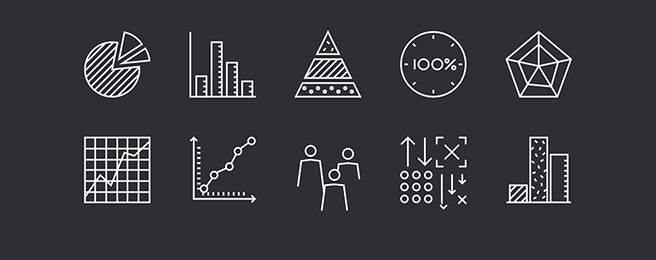Source: https://aperiodical.com/tag/statistics/

Have you ever wondered how combining weak predictors can yield a strong predictor? Ensemble Learning is the answer! This is the first of a pair of articles in which I will explore ensemble learning and bootstrapping, both the theoretical basics and real-life use cases. Let’s do this!

You can look at the code on my GitHub:

### Bootstrapping

Bootstrapping is a resampling method where observations are drawn from a sample with replacement. Let’s say you have 1,000 data points, and you create 100 distinct samples of 1,000 data points each by drawing from the original sample only, with replacement. In practice, this means that there will almost definitely be some duplicate data points in your samples.

To understand why bootstrapping is a powerful tool, one should know a little about statistical inference, the art and science of drawing relevant conclusions for a population based on a random sample of it. With the help of bootstrapping we can obtain an empirical distribution of the value we would like to estimate rather than a point estimate. Why is this important in terms of statistical inference? We’ll see in a minute.

And the importance of this technique goes beyond old-school statistics! When you practice machine learning, one of the first things to do is to split your data into a test and a training data set (and, possibly, a validation set). You fit your model on the training data, and evaluate the results on the test data. This is definitely inspired by statistical inference. The aim of this — as y’all know — is to test the out-of-sample fit of your model — that is, how your model performs on data it did not encounter during training. Or, to put it another way: you’re testing if your conclusions (model) drawn from the sample (fit on the training data) is also valid for the population (represented by the test set).

What bootstrapping achieves is to repeatedly mimic the process of sampling from the population. Resampling from the original sample is just like sampling from the population. Let’s say we draw a 100 samples from 1,000 data points with replacement.

Now, from these 100 samples, one would like to estimate the mean of the population — of which our 1,000 data points are a sample. By calculating the mean of each of the 100 resampled 1000 data points, one achieves an empirical distribution of sample means. Our best guess for the population mean would be the mean of this empirical distribution. The additional information we gain by bootstrapping here — compared to calculating the mean of the sample of 1,000 data points — are the other characteristics of this obtained empirical distribution of sample means.

### And how is this going to be useful for me?

Let’s start with an easy example! Imagine you are the Lead Data Scientist of a company offering some subscription service. One day, the Chief Marketing Officer comes up with an idea: let’s change the landing page of your website, and put a big red flashing SUBSCRIBE button on top of the page. You don’t really believe this is a great idea (who would blame you?), but you ought to be able to come up with some statistics to prove this.

So you design an A/B Test. Half of the users get the old landing page (control group), while the other half lands on the page with the new button (treatment group). After collecting the data of 5,000 users in each group, you can revert to the old page and evaluate your experiment. Let’s assume that normally 1% of visitors subscribe, and that the new button halves the chance of subscribing in general. In the example we simulate data according to this.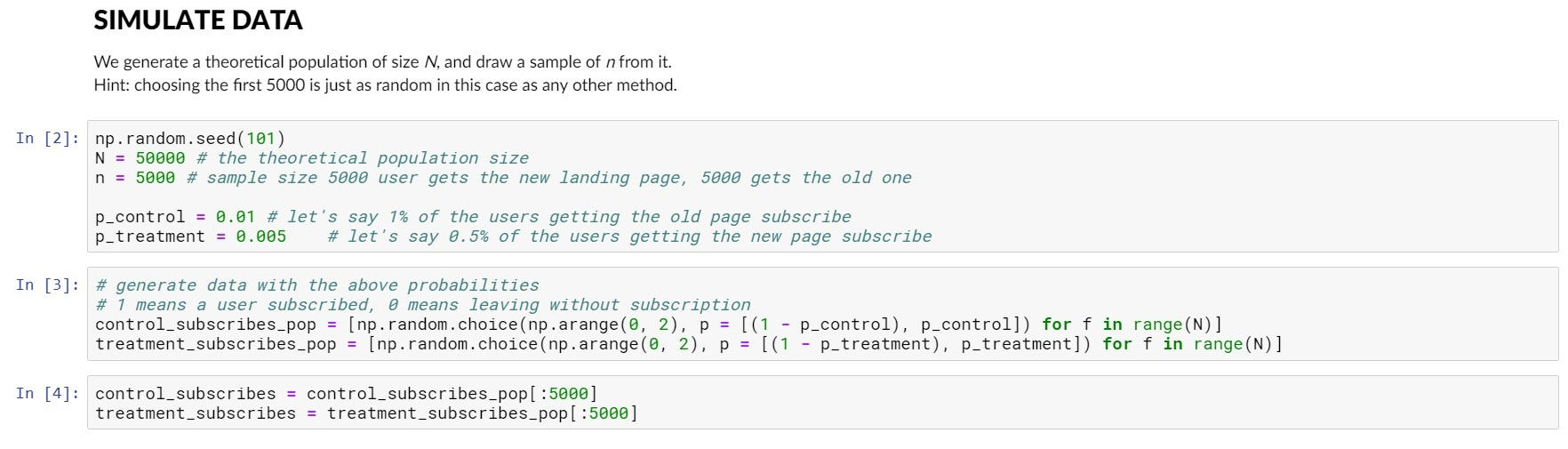Simulation of the population of treatment and control group, then sample from it.

Calculating the mean of these samples would give an unbiased estimator of the probability of subscription on each of the different landing pages. But you need something more: you need to quantify uncertainty in order to provide the bombshell evidence you need to destroy the new SUBSCRIBE button. Without this, you could only give a point estimate about the 10,000 users who took part in the A/B test, and nothing about how representative this value may be for the whole population.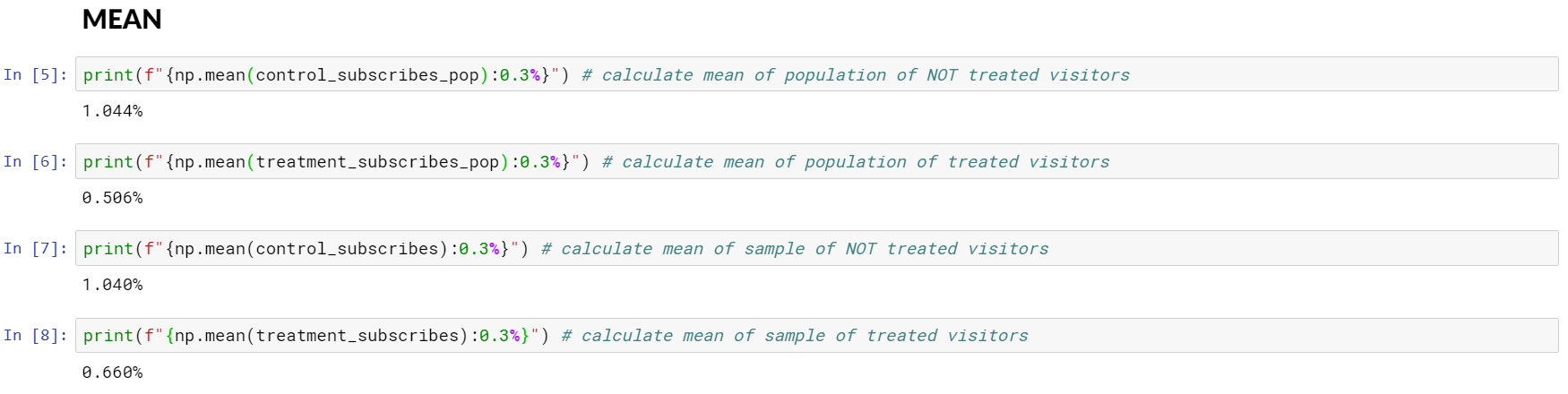Calculating theoretical population and empirical sample means.

So you decide to perform bootstrapping. After performing resampling with replacement 5,000 times from both sets of user data, you end up with a distribution of 5,000 sample means for each user cohort. From this distribution, you can calculate the mean value, and by way of percentiles of this distribution, the boundaries of the confidence interval bounds.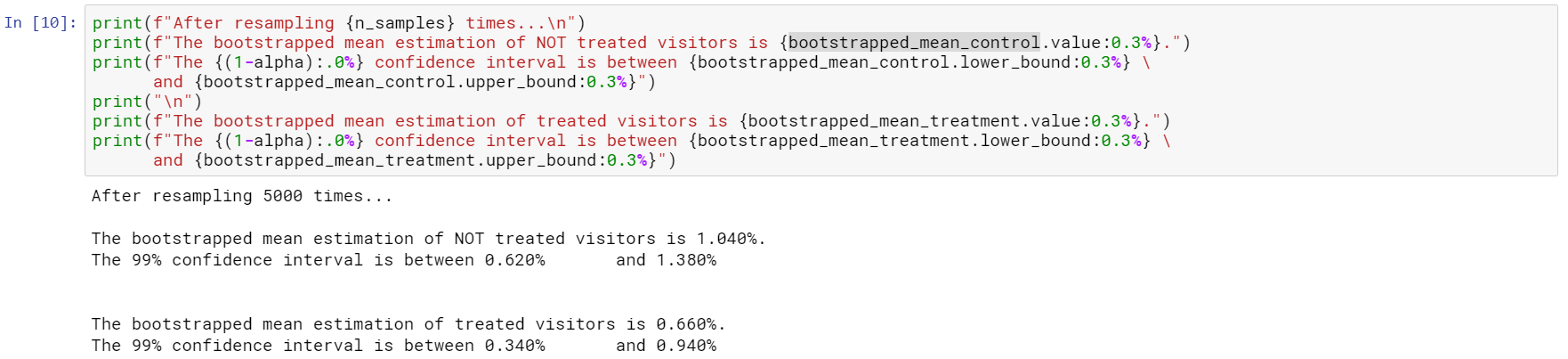Perform bootstrapping and estimate mean from the empirical sample mean distribution.

But you feel you want even stronger evidence, as the lower bound of the 99% confidence interval of the control mean is overlapping with the upper bound of the same interval for the treated group. The following plot is showing exactly this.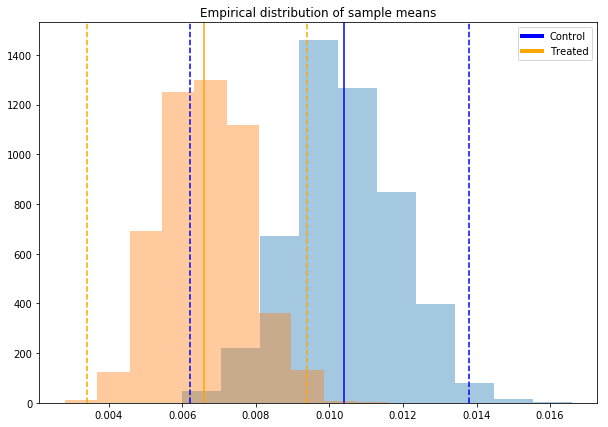Sample means for treatment and control group with 99% confidence intervals.

Luckily, you have a distribution of sample means, and you know how to test equality of means of two samples properly. Yes, yes — two sample t-test it is! This is a statistical test for properly testing the equality of means of two distributions. The final result is a p-value, which is the probability of the null hypothesis (H0) being true. In hypothesis testing, one wants a very low p-value, as this way the null hypothesis is definitely not true — and this is a definitive result.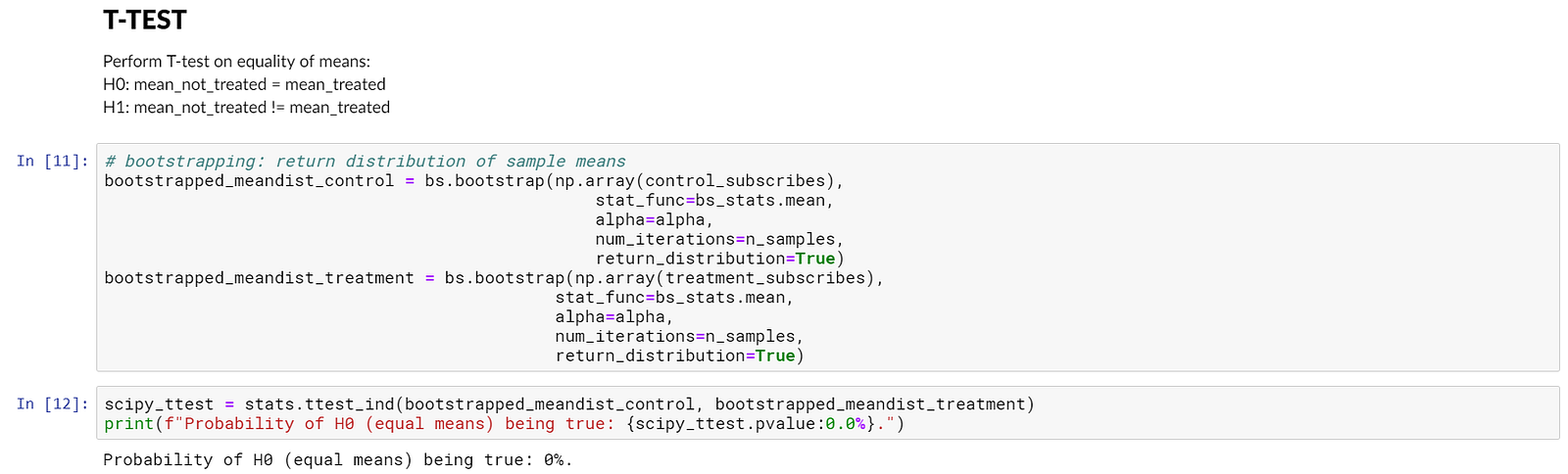Perform t-test for equality of means using scipy.

### Takeaways

BOOM. You just destroyed an idea you didn’t like with a data-driven argument. The CMO cannot say anything but is forced to applaud slowly. You win.

Part 1 of this pair of articles on bootstrapping, boosting and bagging tried to convey the basic idea of bootstrapping, and its main benefit, namely to derive a distribution rather than a point estimate from a data set. This is important, because in this way statistical tests can be done on the resulting distribution, and the uncertainty (sample mean vs mean of sample mean distribution) can be quantified. Stay tuned for part 2, where we encounter the concept of bagging and boosting, along with how they can significantly improve model performance.

Part 2 about bagging and boosting available here!